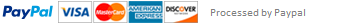### Black-Scholes Option Pricing - Python for Finance

The module should contain a class `SimpleOption` that will satisfy the following documentation. The option class here is super trivial, but it demonstrates an architectural approach: encapsulate assets via their contract terms, and pass that to pricing and risk functions.  A slightly more advanced approach would have a similar data container for the "market environment" (prices, risk free rate, volatilities...) that would also be passed to those functions.

```

class SimpleOption

Simple option contract class capturing the key features of a put or call option. This is just a simple data container.

Parameters

----------

strike : float

strike price for option

is_call : boolean, default True

flag for indicating if the option is a put or a call.

True for call, False for put

Attributes

----------

strike : float

strike price for option

is_call : boolean

flag for indicating if the option is a put or a call.

True for call, False for put

```

The module should also contain the three following methods, and should satisfy the following documentation:

```

bs_price(option, spot, ttm, vol, rate)

Black-Scholes option price for the given market values.

Parameters

----------

option : SimpleOption

The option to price

spot: float

Current spot price of underlying stock

ttm : float

remaining time to maturity in years

vol : float

annualized volatility of the option's underlying stock

15% would be passed as 5.0 and not 0.15

rate : float

annualized riskfree interest rate, as a percent

5% would be passed as 5.0 and not 0.05

Returns

-------

price : float

The Black-Schole price for this option

bs_implied_vol(option, spot, ttm, price, rate)

Black-Scholes implied volatility for the given market values.

Parameters

----------

option : SimpleOption

The option to price

spot: float

Current spot price of underlying stock

ttm : float

remaining time to maturity in years

price : float

current price of option

rate : float

annualized riskfree interest rate, as a percent

5% would be passed as 5.0 and not 0.05

Returns

-------

vol : float

The Black-Schole implied volatility for this option

bs_greeks(option, spot, ttm, vol, rate)

Black-Scholes "greeks" for the given values.

Parameters

----------

option : SimpleOption

The option underlying the calculations

spot: float

Current spot price of underlying stock

ttm : float

remaining time to maturity in years

vol : float

annualized volatility of the option's underlying stock

15% would be passed as 5.0 and not 0.15

rate : float

annualized riskfree interest rate, as a percent

5% would be passed as 5.0 and not 0.05

Returns

-------

(delta, vega, theta, gamma) : tuple(float)

The Black-Scholes sensitivities to various parameters.

delta : sensitivity to change in stock price

vega : sensitivity to change in volatility

theta : instantaneous "time-decay" sensitivity

gamma : sensitivity of the option delta to change in stock price

```

## Basic tests

* A call with zero time-to-maturity has value max(0, price-strike), and a put with zero ttm has value max(0, strike-price).

* Put-call parity implies that \$\Delta(call) - \Delta(put) = 1\$ for a put and a call written against the same stock having the same strike and maturity (under the same market assumptions about volatitility and the risk-free rate, naturally).

This assignment has been answered 5 times in private sessions.